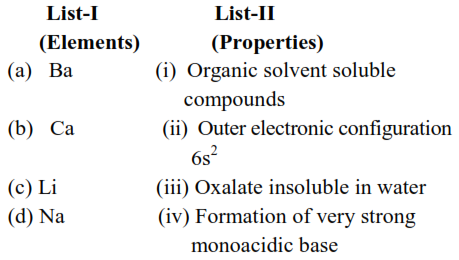# Match List-I with List-II`
Question:

Match List-I with List-IIChoose the correct answer from the options given below :

1. (a)-(ii), (b)-(iii), (c)-(i) and (d)-(iv)

2. (a)-(iv), (b)-(i), (c)-(ii) and (d)-(iii)

3. (a)-(iii), (b)-(ii), (c)-(iv) and (d)-(i)

4. (a)-(i), (b)-(iv), (c)-(ii) and (d)-(iii)

Correct Option: 1

Solution:

(a) 'Ba' having outer electronic configuration $6 \mathrm{~s}^{2}$.

(b) $\mathrm{CaC}_{2} \mathrm{O}_{4}$ is water insoluble

(c) 'Li' is soluble in organic solvents

(d) $\mathrm{NaOH}$ is strong Monoacidic base among given.